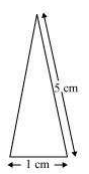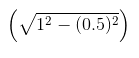Guru

# Radha made a picture of an aeroplane with coloured paper as shown in Fig 12.15. Find the total area of the paper used. Q.3

• 0

Hello sir i want to know the best solution of the question from exercise 12.2  of math of Heron’s Formula chapter of class 9th give me the best and easy for solving this question how i solve it of question no.3 Radha made a picture of an aeroplane with coloured paper as shown in Fig 12.15. Find the total area of the paper used.

Share

1. For the triangle I section:It is an isosceles triangle and the sides are 5 cm, 1 cm and 5 cm

Perimeter = 5+5+1 = 11 cm

So, semi perimeter = 11/2 cm = 5.5 cm

Using Heron’s formula,

Area = √[s(s-a)(s-b)(s-c)]

= √[5.5(5.5- 5)(5.5-5)(5.5-1)] cm2

= √[5.5×0.5×0.5×4.5] cm2

= 0.75√11 cm2

= 0.75 × 3.317cm2

= 2.488cm2 (approx)

This quadrilateral is a rectangle with length and breadth as 6.5 cm and 1 cm respectively.

∴ Area = 6.5×1 cm2=6.5 cm2

It is a trapezoid with 2 sides as 1 cm each and the third side as 2 cm.

Area of the trapezoid = Area of the parallelogram + Area of the equilateral triangle

The perpendicular height of the parallelogram will be= 0.86 cm

And, the area of the equilateral triangle will be (√3/4×a2) = 0.43

∴ Area of the trapezoid = 0.86+0.43 = 1.3 cm2 (approximately).

For triangle IV and V:

These triangles are 2 congruent right angled triangles having the base as 6 cm and height 1.5 cm

Area triangles IV and V = 2×(½×6×1.5) cm2 = 9 cm2

So, the total area of the paper used = (2.488+6.5+1.3+9) cm2 = 19.3 cm2

• 0